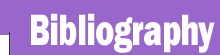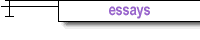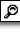Subtle Connection: Non-Locality, Bell's Theorem, Coherent States and the Form of the Wave Function

To take a one week course with David Peat

Abstract

Non-locality in quantum theory is discussed in terms of the global form of the wave function, and as subtle set of necessary and sufficient conditions on the 2-matrix or reduced density matrix. In addition to manifesting itself through the well known Bell's inequalities non-locality also appears as a macroscopic coherence length in condensed and coherent systems. By examining the structure of the 2-matrix, a connection between these two forms of non-locality is made. It is suggested that subtle enfolded orders and non-local forms may have a wider implication and be relevant for a variety of living systems.

Introduction

A distinguishing aspect of the quantum mechanical description of nature is its nonlocality. Bohr, for example, stressed the undivided wholeness inherent in the quantum mechanical description of nature. The essential feature of the double slit experiment is a sort: of wholeness in which changes made at one slit, located a macroscopic distance from the other, result in overall changes to the interference pattern.

The non-locality inherent in a pair of initially correlated particles that become separated by a macroscopic distance has been investigated by Bell1 and in a variety of experiments.2 These experiments demonstrate a non-local correlation that cannot be explained with reference to any "local" theory in classical physics.

This non-classical aspect of quantum theory, its essential wholeness, is also connected to what could be called the "form" of the wave function. In classical systems it is generally possible to separate the system's description into that of various spatially separate subsystems in interaction. In quantum theory, however, the wave function is not spatially separable in this way, into a product of subsystems, and so the special form of the wave function plays a significant role.

While a quantum mechanical wave function is not truly separable into a simple product of different contributions it is, to a good approximation, possible to treat a system of, for example, two alpha particles and four electrons, as being more or less reducible to a description of two helium atoms with a weak interaction between them. However, in certain other circumstances a collection of helium atoms--alpha particles and electrons--will act in a markedly different way, as a single collective or coherent state--a superfluid. Likewise, under certain conditions, a gas of electrons will also act as a single coherent whole.

The nature of these coherent and condensed states is reflected in the very special forms of their wave functions and the fact that they preserve their internal correlations over macroscopic distances. This feature is totally novel to the quantum theory and does not occur in any classical system. Thus form of the wave function exercises a sort of dynamical holism over the system, ensuring the active, dynamical correlation of every particle within the coherent system, no matter how far apart they may be from each other.

This novel dynamical order will be discussed using a formalism known as density matrices, which make certain quantum mechanical implications quite explicit. It will also be used to explore the relationship between dynamical forms and non-locality, or what could be called the "acausal connectivity" inherent in quantum theory.

Quantum Inseparability

Think of two "classical" particles 1 and 2 located at some great distance from each other. The first particle is in state A, say, while the other is in another state B. As the particles slowly approach each other they begin to experience each other's interaction. This is extremely weak at first, since the strength of a force falls off with the inverse square of the distance.

If we ask for the total state S of this weakly interacting system then it is a simple matter to write it as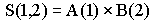This illustrates the essential mechanical nature of classical systems, that they are composed of interacting parts and, in consequence, can be broken down into components again.

Now let us contrast the above description to that of a quantum system where two electrons 1 and 2 are separated by a great distance. By analogy, we would say that the first electron is in stateand the second in state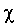. Suppose that they are so far apart that the electromagnetic interaction between them is extremely weak. One would assume that the total state of the system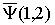could be written, by analogy, asBut this turns out to be entirely incorrect, for the wave function of a quantum system is never separable in this way; it is never reducible to a simple product of states. Indeed, as Neils Bohr so often stressed, quantum systems must always be considered as undivided wholes,

One reason for this essential quantum mechanical non-separability was discovered by Wolfgang Pauli in terms of a special non-local symmetry that must be obeyed by all quantum mechanical wave functions. In essence, for an N-particle system: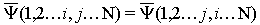or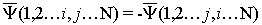That is, under the interchange of any pair of particles the wave function is either symmetric, or antisymmetric. The former case refers to Bose particles (force carriers like photons, gravitons, some mesons, etc.) while the latter refers to Fermions (matter particles like electrons, protons, neutrons, etc.) [More generally, if spin and other internal coordinates are separated out of the wave function then its spatial form must conform to a particular Young Tableau.]

It turns out that, as a result of this requirement of symmetry or antisymmetry, the wave function of a system can never have a separable form, a fact which has a profound theoretical outcome as Bell's Theorem.

Bell's Theorem

The spectrum of ideas leading to Bell's Theorem began with Einstein's attempt to find an objective meaning to the local properties of a quantum system. While Einstein was willing to admit that it may never be empirically possible to specify exactly both the position and momentum of a particle, he nevertheless maintained that such a concept retains an objective meaning. The electron has a path, argued Einstein, even if we are forbidden from ever determining its parameters.

To support this concept of local reality Einstein, along with Podolski and Rosen (EPR), proposed a special type of non-destructive experiment.4 In essence, an initially correlated system is separated into two correlated parts. Since the overall momentum of the system is conserved it should be possible, by measuring the momentum of one particle to deduce the momentum of the other. Likewise, a measurement of the position of one particle enables us to deduce the position of the other. Thus, Einstein believed, it is meaningful to describe the undisturbed electron as having well-defined properties--position and momentum. By never observing the particle directly and only making measurements on its correlated partner the election's independent reality was never disturbed.

Bohr5 however, countered the EPR argument by stressing the holistic nature of quantum systems and suggesting that the very disposition to measure one of a pair of non-commuting observables changes the whole context of an experiment and renders the meaning of the second observable ambiguous.

A further step came with Bohm6 who focused the argument on the antisymmetry of the wave function. A pair of particles in an eigenfunction of an antisymmetry operator will continue to remain in such a state no matter how far apart they separate. The overall antisymmetry of the wave function for the two electrons has nothing to do with any physical interaction between them. Indeed, this non-local property is essentially non-classical in nature. Such non-locality is incompatible with a theory based on the notion of a local reality or any local hidden-variable theory.

It remained to John Bell to demonstrate how non-classical correlations between pairs of particles, arising out of the (non-local) antisymmetry of the overall wave function, can be experimentally and definitively distinguished from the predictions of a local reality theory. Such experiments have been repeated many times and come down firmly in favor of non-local, quantum mechanical reality.

The special dynamical form of the wave function, called antisymmetry, is the essential feature leading to non-separability and the sort of "acausal" connection described by John Bell. The implications of Bell's Theorem have led to all manner of speculation. What, some people have speculated, would be the effects of non-local correlations within living systems and, in particular, the brain! There have even been attempts to explain such things as telepathy and the paranormal in terms of Bell correlations, i.e., through correlations that do not fall off with distance. However, these discussions generally miss the important point that the Bell correlations are not mechanical in nature, they do not involve, for example, some new sort of instantaneous force. It is no use attempting to construct a new sort of long range transmitter, or telepathic device, that would make use of Bell correlations to transmit information from one location to the next. What Bell's Theorem demonstrates is an essential wholeness within the quantum system and a correlation of its total state that can only be understood in non-local terms.

Now cautioning us to avoid seeing the Bell correlations in terms of forces and mechanical connections does not mean that non-locality may not have important implications elsewhere. Indeed, as we shall see, the global nature of the wave function plays a key role in all coherent systems. Bell's famous theorem, and the active discussion that still surrounds it, has always been concerned with the non-classical correlation of only two particles. But, as this appendix paper shows, this dynamical, non-local correlation is generally true of all quantum states. In the discussion of subtle energy processes the whole notion of global form was emphasized. Now we see how one such example of a global and non-local form arises out of the antisymmetry of the wave function. This antisymmetry, and the significance of the form and the wave function is an essential feature of quantum theory. In the sections below, some of the implications of these quantum connections will be demonstrated using a particular formalism, called the Density Matrix, to render them explicit.

The Density Matrix

The wave function is the usual descriptive form for quantum theory. However, the so called reduced density matrix, although less well known, can also be used as a quantum mechanical description and, moreover, contains the Pauli antisymmetry (or symmetry) conditions in a particularly interesting enfolded form. Using the 2-matrix, therefore, non-local correlations and antisymmetry can be displayed in a new formalism. The reason for employing the density matrix formalism in this paper is simply to express the notion of "form" in a new way, one that may suggest novel approaches.

The formalism that is relevant for considering these correlations is called the second order reduced density matrix, or Z-matrix. It is obtained by integrating over an N-particle wave function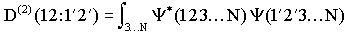(1)

In a sense, a certain degree of information is lost within this process of integration. However, valuable information remains, in an enfolded form, within the 2-matrix and therefore knowledge of this matrix alone is sufficient to allow any expectation value of the N-particle system to be calculated exactly. What is of particular interest is how the Pauli conditions are reflected in the form of the matrix.

First let us see how critical information about the quantum system has been enfolded into the 2-matrix. One would normally calculate the energy of an N electron quantum system in the following way(2)

where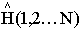is the Hamiltonian of the system(3)

In terms of the 2-matrix this energy can now be written exactly as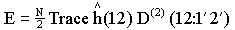(4)

where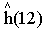is called the reduced Hamiltonian(5)

And, let me stress, that (4) is not an approximation but an exact result.

This reduced Hamiltonian has a spectrum of eigenvalues and eigenfunctions(6)

We can expand the 2-matrix in this complete set of eigenfunctions(7)

This enables expression (4) for the energy to be written as(8)

which means that in order to calculate the total energy of the N-electron system all we need to know is the complete solution to a corresponding 2-electron problem (the eigenvalues to the reduced Hamiltonian) together with the coefficients {Bnn}. This is a very interesting conclusion, for it tells us that all the complexity of the many body problem is enfolded within the solution of a corresponding 2-body problem (using the reduced Hamiltonian) plus a set of the numerical coefficients. It turns out however that the {Bnm} contain, in an enfolded way, all the subtle information about the various global correlations within the quantum many body system.

This result holds for any observable or measurement within the system. All one needs to know are a set of eigenvalues to the reduced two-body operator and the corresponding numerical coefficients in the 2-matrix.

It seems that the many body problem has been partitioned into two parts, one of which deals with a paradigm two body problem and the other (the {Bnm}) of which contains information about different sorts of correlations between electron pairs within the system, including those non-local correlations that are the direct result of Pauli's principle. (This is not to say, however, that the quantum system has been physically partitioned; rather, the mathematical formalism for representing the various correlations within the system.)

It turns out that what is physically really interesting about a many electron system, or other many body problem, is present in this second part, that is in the complex and subtle interrelationships amongst the {Bnm} for it is here that the implications of the non-local form of the wave function have hidden themselves.

As an example, suppose we wish to determine the energy of a large atom, The first step would be to calculate the complete set of two body energies and eigenfunctions to the reduced Hamiltonian. This can be done in a fairly straightforward fashion to a high degree of approximation.

Already a great deal of information is contained within this complete set of eigenvalues so one may perhaps be excused for believing that the difficult work of calculating the total energy had already been done. One would assume that these values would be inserted into equation (8) along with some reasonable assumptions for the values of each of the {Bnn}. Very quickly, however, one learns that this approach gives disastrous results.

In fact, even by imposing some very reasonable constraints upon the {Bnn} the calculated energy falls far below the true ground state energy for the atom. This indicates that the Variation Principle has been violated--in other words, that one must have been working with a 2-matrix that is not derivable from an antisymmetric wave function. (The Variation Principle states that energies calculated with any trial or approximate wave function will always lie above the true energy of the system. One normally proceeds by making constant adjustments to this trial wave function until the energy falls as low as possible--i.e., approaches the true energy asymptotically from above. It is only possible to violate this principle if the wave function does not possess the correct antisymmetric form.)

We are therefore faced with the following quandary. The 2-matrix allows us to calculate, in a very compact form, the expectation value of all quantum mechanical observables for a particular system. All we have to ensure is that a trial Z-matrix should be derivable from a wave function that has the correct antisymmetric form. The conditions that would correspond to this restriction are called the N-representability conditions and, it turns out, that they are extremely subtle for they express what could be called an enfolded relationship between the complete set of coefficients {Bnm} and the eigenfunctions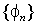to the reduced Hamiltonian.

Despite considerable research activity these conditions are not known in an explicit, or what for a better word could be called a compact, form. Rather one must always first go to a full N-particle wave function in order to impose conditions on the 2-matrix. The goal of N-Representability conditions would be to discover some new conditions for dynamical non-locality, upon the 2-matrix. Clearly this must occur in nature in a very interesting and subtle way. Indeed, the full import of the special form, or non-locality of the wave function can only be appreciated when one goes to the 2-matrix. While Bell's Theorem was specifically formulated to deal with the non-classical correlation of only two electrons one can see within the Z-matrix a more general set of conditions that express the non-classical correlations of a many body system.

Atomic Correlations

Let us look at a simple example, an atom which contains four electrons. Since physical systems seek the lowest possible energy one would assume that, to a good approximation, one would simply inset the lowest eigenvalues en, in equation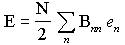(9)

with appropriate bounds upon the Bnn. It can be shown that these bounds are of the form(10)

where N in this case is 4, i.e., the Bnn, must all be less than or equal to 1/3. So as a first guess one would approximate the total energy of the system using the first three 2-electron energy levels, i.e., 1s2, ls2s 1S and ls2s 3S with equal weights of 1/3. However, as we have hinted above, this gives an absurdly low and physically unacceptable result. In fact, the antisymmetric form of the wave function demands that an additional 2s2 energy level be included in (9). What is striking about this energy level is that it lies within the 1sns continuum--i.e., there are an infinite number of 2-particle energy levels lying below it. Nevertheless what could be called the antisymmetric form of the wave function, or its non-local correlations, are enfolded within the 2-matrix in such a way that the Bnn are not independent of each other but are all related through a set of conditions enfolded within the eigenfunctions.· Specifically at this first level of approximation:

 B11 = 1/6 1S B22 = 1/6 1S B33 - B55 = 1/6 3S (triply degenerate states) B66 = 1/6 2S
(11)

I cannot stress too much the importance of this result, that a 2s2 level should be included. It demonstrates that antisymmetry--the non-local form of the wave function--makes an unusual and non-classical demand upon the 2-matrix, a demand that forces upon it an enfolded order.

Why do we call this a non-local effect? Because the special form of the wave function, its antisymmetry, has a profound effect not only upon the dynamics of each electron but upon the way in which the electrons interact with each other. Let me illustrate this in the following way. Suppose, in the above example, one were to switch off the electromagnetic interactions between electrons, retaining only the attractive force between each electron and the atomic nucleus.

The Pauli principle dictates that rather than electron pairs all occupying the lowest energy 1s state, they must arrange themselves according to expression (11), i.e., pairs of electrons also occupy the ls2s and 2s2 states.

In itself this is a non-classical result. Now look what happens when the electron-electron interaction is switched on in a progressive way. Suppose at a first approximation we allow each electron, in turn, to experience the electron density of all the others, i.e., to move in the electrostatic field produced when the other electrons are held in place. By repeating this process successively with each electron, one can investigate the average effect of how each electron adjusts to the interaction of all the others. This is what is known as the Hartree-Fock approximation and, using the wave function approach, it involves writing the wave function as a single Slater determinant and varying each electron orbital until the total energy is minimized.

What is the equivalent approach in the 2-matrix picture? The Hartree-Fock approximation is exactly the same as switching on the full electron-electron interaction within the reduced Hamiltonianand determining its exact energy eigenvalues. That is, the Hartree-Fock energy is equivalent to solving the interactive 2-electron problem exactly. However, in writing down the expression (9) for the total energy one still retains the same set of first approximation {Bnn} given by expression (11). In other words, the particularly weighting factors associated with each energy eigenvalue are those calculated in the absence of electron-electron interaction. That is, while they include the Pauli requirements at what could be called the first level of approximation, they do not take into account the very fine details of an electron pair's dynamics as it responds to the detailed interaction of all other electrons.

In a sense, however, nothing truly unexpected has happened. The real crunch, the truly exciting physics, happens when the final amount of the electron-electron interaction is fully switched on and one is forced to move beyond the Hartree-Fock approximation. In the Hartree-Fock approximation each electron was allowed to adjust to the average motion of all the others. Or, in terms of the 2-matrix, while the exact energy eigenvalues for the 2-body problem were used the weighting factors {Bnn} did not take into account the detailed changes within dynamics of all the electrons.

It turns out that under the full electron-electron interaction one must take into account the full nature of the eigenfunctions to(also called geminals). This is how the special form of the many body wave function is enfolded within the 2-matrix, for the effect of making a change to any one of thewould affect the entire 2-matrix. In other words, each weight factor Bnn is linked in an enfolded way to all the other {Bnm} and to the complete set of. So an interaction that makes even a small change to one particular geminal will effect the whole 2-matrix by changing all the weighting factors {Bnm}.· Or, to put it another way, the dynamics of every pair of electrons is correlated in an enfolded or non-local way to every other pair. Earlier it was suggested that, within the Density matrix formalism, the many body problem had been partitioned into two parts-one dealing with a paradigm two body problem and the other giving the {Bnm}· But now we see that a subtle relationship exists between theand the {Bnm}. This relationship is related to the overall global form of the wave function. Or, to put it another way, the subtle internal order of the density matrix arises out of the essential non-local form of the entire quantum system.

To transform from a full wave function into a 2-matrix involves a process of mathematical integration, which is really a sort of enfoldment. In this way, the special form of the wave function is enfolded into a subtle set of mutually dependent conditions that link together all the pair functions and weight factors. What this means, in effect, is that each electron pair is correlated in a very subtle way with every other electron pair. And this correlation, moreover, is not simply the result of the physical force of electromagnetism but a sort of enfoldment of two effects--electromagnetic interaction and N-representability (i.e., the requirement of antisymmetry for the total wave function).

This, I believe, is the full importance of what was only implicit within Bell's result. Because of the non-separability of the general N-electron wave functions, the motion of each electron is related to the whole, not simply through a physical force but through non-local correlations.9 And so the fine details of the physical interactions between electrons is experienced within the whole system. To borrow David Bohm's phrase, the antisymmetry principle really manifests itself within the implicate order, for it enfolds in a subtle fashion restrictions and correlations upon the electron dynamics.

In this way, I believe, one can establish a metaphoric connection between non-locality, dynamical form and discussions of subtle energy. With the Bell correlations and the N-representability conditions on the 2-matrix we have a subtle form of non-locality that exerts a correlating effect on all parts of the quantum system. One may, perhaps, wish to speak of a correlated dynamics within the system. However, these very conditions caution us that the system is an indivisible whole and should not be treated as composed of even correlated parts. This non-local holism may indeed have significance for a consideration of subtle energy within living systems; it certainly does so for coherent and condensed matter.

But this is not to say that one would necessarily use the 2-matrix language in order to discuss subtle energy. Indeed the drawback of this form of description is its somewhat static nature. Rather the purpose of this appendix is to explore the implications of "enfolded non-locality" and "global form" within a particular formal language. By showing the particular subtle way in which this dynamical order operates one may perhaps gain a new respect for subtle process and of the need for a new formal language in which to discuss non-local correlations and the circulation of subtle energy.9

Superconductivity

A novel feature of quantum theory is that the wave function must have a special overall form and, as we have shown above, one can also see this in an equivalent way as the enfolded order of the 2-matrix. It turns out that the effects of this enfolded order are even more remarkable when it comes to superconductivity and correlated systems. Indeed, it becomes apparent that the very phenomena are a direct result of this enfolded order, i.e., that such coherence and global order is not possible for classical systems.

Consider the case of a BCS superconductor. (We will leave aside the mysterious details of the new High Temperature superconductors.) The conventional BCS theory has it that a phonon-mediated10 interaction causes a coupling between electrons in momentum space--the Cooper pairs. In most accounts of superconductivity it is held that the entire electron gas condenses into these Cooper pairs, i.e., that the pairs behave as Bosons and an actual Bose-Einstein condensation occurs. It is this supposed condensation which is then held responsible for the phenomenon of superconductivity.

This, in fact, is not correct and the actual collective state is the result of a much more subtle tension, or enfoldment, between these electron pairs and the overall antisymmetric form of the wave function or, in the case of the 2-matrix, the N-Representability conditions. As we shall see, within this tension of N-Representability, it is possible for the 2-matrix to take on a special form in which off-diagonal long range order (ODLRO) is present so that correlations persist across macroscopic distances. These macroscopic correlations are in fact common to all coherent systems and are particular feature of quantum theory with its curious non-locality.

Again we only begin to see the true nature of the constraints of overall dynamical form when we come to work within the 2-matrix formalism. Normally a discussion of superconductivity would take place within a second quantized formalism, and while this may be convenient for numerical calculations it obscures other details and leads, for example, to the erroneous conclusion that Bose-Einstein condensation is possible within an electron gas.

For the purpose of the present discussion let us work with diagonal form for the 2-matrix(12)

Since

theare eigenvalues to, with the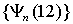as corresponding eigenfunctions. In general, for electrons in an atom, molecule or metal the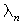, are of the order of 1/N2 with, as was shown in the previous section, a subtle enfolded relationship between the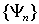and the eigenvalues.

However, a general result that follows from the basic requirement that a 2-matrix should be N-Representable (i.e., a necessary but not sufficient condition that it should be derivable from any general sort of antisymmetric wave function) is that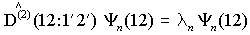(13)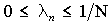(14)

As was said before, this upper bound is never normally approached. However, in the case of a superconductor it turns out that(15)

along with of the order of N2 additional states, each with very small eigenvalue of the order of 1/N2.

The occupation number of the lowest state, the Cooper Pair, is therefore not 1, as is normally believed, but of the order of 1/N. Condition (15) is an unavoidable restriction, a limit that nature places upon the internal dynamics of all fermion systems, no matter how they chose to pair themselves up. We therefore see that, thanks to condition (15) which is a particular expression of the Pauli Principle, the expectation value of the Cooper pair can never exceed 1/N.

Not only are the statistics of electron pairs not Bose-Einstein, neither are they what are called "intermediate statistics". It is sometimes incorrectly held that, through the action of certain attractive forces, electron pairs obey a form of statistics that lies midway between Fermi-Dirac and Bose-Einstein. In such a case thewould be represented as a set of variables whose bounds lie between the occupation numbers corresponding to Bose and Fermion pairs and each of which is a simple function of the others. However, the true statistics of these occupation numbers is far more complicated than this because each is dependent, in a complicated way upon all the other occupation numbers and eigenfunctions of the 2-matrix.

These considerations show us that the statistics of electron pairs within a condensed system are indeed far more complex than Bose-Einstein or Intermediate statistics. Indeed, it is the overall form of the wave function, or the subtle N-Representability conditions, that give rise to the coherent behavior within a superconductor whereby the dynamics of the electrons are interconnected in a very complex fashion.

In particular, C.N. Yang8 has shown that, despite the fact that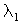is vanishingly small, there will still be off-diagonal long range order within the 2-matrix. In other words, thanks to the subtly enfolded order of the 2-matrix, the correlation between different regions of the superconducting electron gas does not vanish at macroscopic distances.

### Superfluidity

A discussion of superfluidity in terms of the reduced density matrix is far more complicated than in the case of a superconducting electron gas. Most texts treat the helium atoms in a superfluid as pure Bose particles and the phenomenon itself as a case of Bose condensation. At a certain level of understanding this may be helpful. However, the simple fact of Bose condensation is not sufficient to produce a superfluid. What is required is a coherent wave function in which scattering into higher momentum states is prevented. Without this additional requirement, a condensed state would not exhibit flow without resistance.

The coherence of the superfluid's wave function arises in the attractive interaction between helium atoms as a result of their polarization. A proper treatment of a superfluid must therefore include virtual excitations of the electronic wave functions around each helium atom, a fact that immediately leads to an extremely complicated statistics for the helium atoms themselves--a statistics which does not simply deviate from pure Bose statistics into "intermediate statistics" but involves the sorts of enfolded conditions met in the case of superconductivity.

A deeper insight into this problem would involve detailed analysis of the reduced density matrix as a function of both the nuclear center of mass and electronic coordinates. The N-Representability conditions on such a matrix would then arise from symmetry in nuclear center of mass coordinates and antisymmetry in electronic coordinates. This analysis is beyond the scope of the present appendix. However, it is sufficient to note that macroscopic coherence and off-diagonal long range order in the 1-matrix (expressed in terms of nuclear coordinates) follows directly from subtle and complicated interrelationships arising in the higher order density matrix, expressed in both electronic and nuclear coordinates. Again long range order, global symmetry and antisymmetry requirements on the wave function are interrelated.

### Conclusions

The global symmetry or antisymmetry of the wave function is a feature unique to quantum theory. Information enfolded within the form of the wave function plays an important role in both the sort of non-local correlations discussed by John Bell and in the detailed behavior of condensed and coherent systems such as superconductors and superfluids.

What is of particular significance is that coherent states may play a key role in all living systems. Indeed, there may be something essentially quantum mechanical about life. This special feature seems to involve a sort of enfolded order of dynamics, a subtle correlation that enfolds and unfolds within the different movements of the system, Such an order is not inherent within "classical systems" in which such non-local, or global, symmetry requirements are not available.

The importance of the reduced density matrix representation in understanding this is that non-locality in the wave function becomes explicitly translated into a set of enfolded interrelationships between states and occupation numbers. In a condensed state, for example, these correlations ensure off-diagonal long range order which corresponds directly to macroscopic coherence within the physical system. The density matrix approach was therefore used in this appendix in order to illustrate how non-locality can appear us an enfolded or implicate order form. However, in the light of the previous discussions of verbal languages and process mathematics we must acknowledge that, as presented here, the density matrix remains static and does not form to the content of description.

The unique feature of quantum theory, its rejection of local reality, has been emphatically demonstrated by Bell in the particular case of a correlated pair of electrons that are then separated by a macroscopic distance. We have now seen how manifestations of non-locality can also be found within condensed systems and may well be the key to living systems. Subtle correlated changes to the boundary conditions of sensitive systems can produce large, coordinated changes. The mechanism of memory in the brain, for example, while it remains obscure, may well be non-local in nature and it is possible that global correlations of boundary conditions could play a role in the nervous system. Such notions as the circulation of energy, information and subtle correlations throughout the body may well have some connection to some of the ideas discussed in this appendix.

### References and Notes

1. J.S. Bell, Physics 1(1964), p, 195.
2. For example see A. Aspect, A. Dalibard and G. Roger, Physics Review Letters 49 (1982), p, 1804.
3. A review which contains references to the various properties of reduced density matrices discussed in this article can be found in F. David Peat, Physical Chemistry, An Advanced Treatise (H. Eyring, D. Henderson & W. Jost, Eds. (Academic Press, New York, NY, 1975), p· 429.
4. A Einstein, B. Podolsky & N. Rosen, Physics Review 47 (1935), p. 777. See also Quantum Theory and Measurement. J. S. Wheeler and W. H. Zurek, Eds. (Princeton University Press, Princeton, NJ, 1983).
5. N. Bohr, Physics Review 48 (1935), p. 696.
6. D. Bohm, Quantum Theory (Prentice-Hall, Englewood Cliffs, NJ, 1951).
7. A. J. Coleman, Review Modern Physics 35 (1963), p. 668.
8. C. N. Yang, Review Modern Physics 34 (1962), p. 694.
9. A note of caution about that word "correlation": properly speaking it means the connections within a system. However, theoreticians who make calculations of atomic and molecular energies have come to use the word in a more restrictive form, i.e., as "correlation energy," meaning a set of detailed corrections that go beyond the Hartree-Fock approximation. In the present paper, however, the world "correlation" is being used in its more general sense.
10. Phonon (a quantized vibration of the metal lattice).

Related Pages: Science |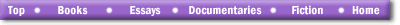Contact F. David Peat